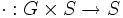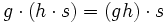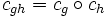Switching between the left and right action conventions

One of the frequent sources of minor confusion in literature using groups is the confusion between the left action convention and the right action convention. In much of group theory, the right action convention is common, while in most of mathematics outside of group theory, the left action convention is prevalent. This article discusses the two conventions, how to translate from one convention to another, and how to show that both conventions are equally strong and no real difference is made in switching from one to the other.

The left action convention versus the right action convention

Functions are written on the left versus on the right

The underpinning of the left action convention is that all functions are written on the left. Thus, to denote the image of an element$x$ under a function$f$, we write$fx$ or$f(x)$.

In the right action convention, functions are written on the right. This need not be the case for all functions, but it is definitely the case for the functions that are obtained through the action of group elements. Thus, the image of an element$x$ under a function$f$ is denoted as$xf$ or$x^f$ (the right-exponentiation notation).

Functions compose right-to-left versus left-to-right

Under the left action convention, applying a function$f$ and then a function$g$ is written as$g \circ f$. In other words, the functions applied later are written on the left. This is because applying$g$ after$f$ to$x$ gives$g(f(x))$, whence$g$ appears on the left.

Under the right action convention, we write$fg$ for applying$f$ first and then$g$, because$(x^f)^g = x^{fg}$ feels more natural.

Group actions on the left versus on the right

Further information: Group action

Corresponding to functions on the left, a group action of a group$G$ on a set$S$ is a map$\cdot:G \times S \to S$ such that$g \cdot (h \cdot s) = (gh) \cdot s$. On the other hand, a group action on the right is a map$\cdot: S \times G \to S$ such that$(s \cdot g) \cdot h = s \cdot (gh)$. Note that under the left action convention, acting by$h$ and then by$g$ is equivalent to acting by$gh$, while under the right action convention, acting by$g$ and then by$h$ is equivalent to acting by$gh$.

Left and right cosets

Further information: left coset, right coset

The left cosets of a subgroup$H$ of a group$G$ are subsets of the form$gH, g \in G$. The right cosets of$H$ in$G$ are subsets of the form$Hg, g \in G$.

There is a double interplay of left and right:

• The left cosets are the orbits of$G$ under the action of$H$ on the right by multiplication.
•$G$ has a left action on the left coset space by left multiplication. Further information: Group acts on left coset space of subgroup by left multiplication
• The right cosets are the orbits of$G$ under the action of$H$ on the left by multiplication.
•$G$ has a right action on the right coset space by right multiplication.

Conjugation

Certain automorphisms of a group, called inner automorphisms, arise via conjugation by elements of the group. Left and right action conventions again play a role in determining what the conjugation by an element$g$ is.

Under the left action convention, conjugation by$g$ is the map$x \mapsto gxg^{-1}$. This is denoted$c_g(x)$ or${}^gx$. This convention is chosen because under this convention, group acts on itself as automorphisms by conjugation. Specifically, with this convention,$c_{gh} = c_g \circ c_h$.

Under the right action convention, conjugation by$g$ is the map$x \mapsto g^{-1}xg$. This is denoted$x^g$. This convention is chosen because under this convention,$x^{gh} = (x^g)^h$. In other words, we need this convention to obtain that the group acts on itself as automorphisms by conjugation if we are using the right action convention for the definition of group action.

Thus, conjugation by$g$ with respect to the left action convention equals conjugation by$g^{-1}$ with respect to the right action convention.

Commutators

Under the left action convention, we define the commutator of two elements as:$[x,y] = xyx^{-1}y^{-1} = xc_y(x)^{-1}$.

Under the right action convention it is defined in two different ways. The typical definition is:$\! [x,y] = x^{-1}y^{-1}xy = x^{-1}x^y$

These different conventions for commutators result in slightly different formulations of formulas such as formula for commutator of element and product of two elements and Witt's identity.

Opposite multiplication for symmetric groups

Since the elements of the symmetric groups are defined as permutations, and composition of permutations is by function composition, the choice of left versus right action conventions leads to opposite rules of multiplication.

Why the conventions are equivalent

The two conventions are equivalent and it is possible to translate from one convention to the other.

Inverse map translates from left to right

Further information: Every group is naturally isomorphic to its opposite group via the inverse map

The opposite group to a group is the group with the same underlying set with the new multiplication defined as the old multiplication with the order of elements reversed. The inverse map from a group to itself is an isomorphism from the group to its opposite group. In other words, reversing the order of multiplication (i.e., switching from left to right) does not change the nature of the group.

The isomorphism is natural in the sense that it commutes with homomorphisms, subgroup inclusions, etc. In other words, doing the inverse map and then applying a homomorphism is equivalent to applying the homomorphism and then doing the inverse map.

Particular rules for translation

• A statement made about a specific element or subset is true if and only if the opposite statement made for the inverse element or the subset of inverses to those elements is true.
• A statement made about a subgroup or about subgroups is true if and only if the opposite statement is true for that subgroup.
• Any statement that quantifies existentially or universally over elements of the group, rather than talking about specific elements, is true if and only if the opposite statement (i.e., the statement obtained by reversing the roles of left and right) is true.

For instance, left and right coset spaces are naturally isomorphic: the space of left cosets of a subgroup$H$ of a group$G$ can be identified with the space of right cosets, via the inverse map.

Most of the statements we make in group theory are made at the level of subgroups, and the primitive concepts used in these statements are robust under left-right interchange. Thus, for most of these statements, it does not matter whether, while proving the statement, we use the left action convention or the right action convention. In other words, a high-level statement about subgroups and their behavior may use terms that have different formulas for left and right action conventions, but the truth of those statements is independent of the formula we choose to use to prove it.

On the other hand, there are some statements, particularly concrete formulas about conjugates and commutators, that do depend on the left/right action convention. In other words, the formula as stated makes sense only in one convention. However, it can usually be translated easily to the other convention.

Equally strong

The left and right action conventions are both equally strong. Any proof in one convention can be translated line by line into a proof in the other convention using the inverse map that establishes the natural isomorphism.

The reason group theorists prefer the right-action convention is largely because of the convenience of right-exponential notation in handling formulas, as well as the fact that left action can then be reserved for other kinds of actions (such as field scalars acting on a$G$-module). In much of the rest of mathematics, the left action convention is the dominant one.

On this wiki

On this wiki, we generally try to make statements that are robust and independent of the action convention. For those statements that are at the level of formulas, we try to state them in both conventions. The default convention used when nothing is stated is the left action convention. For more, see Category:Groupprops conventions.